## Introduction

The genetic architecture of a trait can greatly influence the outcome of genetic drift and selection. In the infinitesimal model, a very large number of genes each with very small additive effects contribute to a trait (Fisher, 1918). When truncation (or divergent) selection is applied to the infinitesimal model, the change in genetic variance is temporary because linkage disequilibrium is induced between the selected genes. As soon as selection ends, genetic variance tends to be restored to the original level (Bulmer, 1985). The number of generations of random mating required to restore the level of genetic variance depends on the degree of linkage disequilibrium in the population (Crow and Kimura, 1970). Conversely, for a finite locus model, changes in genetic variances owing to truncation selection can be permanent as some or even all loci could get fixed for the favorable allele. Recently, the rapid expansion of molecular methods for determination of quantitative trait loci (QTL) has given support for the presence of major genes behind many characters (e.g. Orr, 2001; Barton and Keightley, 2002; Slate, 2005).

Fisher (1918) also emphasized that the genetic variation underlying a quantitative trait could be partitioned into different components using a least-square principle. The partition includes an additive part due to the additive effect of an individual allele, a dominance part due to within-locus interactions, and an epistatic portion due to between-locus interactions. Many different quantitative genetic models have since been proposed to represent the genetic effect on a phenotype. Cockerham (1954) and Kempthorne (1954) proposed a parameterization to interpret how different genetic effects contribute to the genotypic value, including dominance and epistasis. Cockerham (1954) used two parental populations to construct an F2 reference population with two loci in Hardy–Weinberg equilibrium, and derived an orthogonal partition of the genetic variance in the F2 population. Crow and Kimura (1970) and Mather and Jinks (1982) used a two-locus genetic model to examine epistasis, but unlike Cockerham, they used an F reference population (pure lines derived from selfing). While most genetic models are based on Crow and Kimura (1970) and Mather and Jinks (1982), Kao and Zeng (2002) and Zeng et al. (2005) have further extended Cockerham's genetic model to include multi-locus gene interactions in a segregating population for the purpose of QTL analysis.

That the additive genetic variance should decrease in a finite population because of genetic drift is a well-established principle of evolutionary biology (Crow and Kimura, 1970; Falconer and MacKay, 1996). However, a number of publications have shown that this conclusion might be oversimplified if non-additive interactions contribute to trait expression. The increase in additive variance in a population experiencing genetic drift could be explained by both dominance and epistasis (e.g. Goodnight, 1988; Wang et al., 1998; López-Fanjul et al., 2002). Willis and Orr (1993) investigated the role of dominance variance in bottlenecks, where they emphasized that a different degree of dominance could increase the release of additive variance. The conversion of epistatic to additive genetic variance during population bottlenecks has been studied theoretically by Goodnight (1988), Cheverud and Routman (1996), López-Fanjul et al. (2002) and Naciri-Graven and Goudet (2003). Wang et al. (1998) used data on viability in Drosophila melanogaster going through bottlenecks to quantify the effect of dominance, and Waldmann (2001) suggested that high levels of additive variance in a small population of the plant Scabiosa canescens could be explained by the presence of dominance. This phenomenon has also been verified experimentally in the housefly (Bryant et al., 1986; Bryant and Meffert, 1996), in D. melanogaster (López-Fanjul and Villaverde, 1989; García et al., 1994) and in mouse (Cheverud et al., 1999).

Under the finite locus model, when directional selection is acting on a population for a certain quantitative trait, the additive genetic variation is also expected to decrease according to traditional quantitative genetic theory (Crow and Kimura, 1970; Falconer and MacKay, 1996). However, some theoretical studies of selection suggest that the level of additive variance can be sustained or even increased when non-additive variance is present (Gimelfarb 1989; Fuerst et al., 1997; Jannink, 2003; Carter et al., 2005), in a manner similar to the action of genetic drift. Experimental evidence for this phenomenon was found by Martinez et al. (2000) when they selected for body fat in mice, and by Sorensen and Hill (1982) who performed a short-term selection experiment for abdominal bristle number in D. melanogaster. Furthermore, in a recent study, Carlborg et al. (2006) showed that epistatic interactions between four loci mediated a considerably higher response to selection of growth in chicken than predicted by a single-locus model. However, none of these studies have compared the development of variance components between different genetic models in situations of directional selection.

Theoretical investigations of the effect of selection on the re-distribution of additive and non-additive genetic variances are scarcer than for the drift situation. Pair-wise epistatic gene interactions affecting a trait have been simulated for the directional truncation selection process (Gill, 1965a, 1965b and 1965c; Young, 1966, 1967; Fuerst et al., 1997). However, few simulations have been performed on multiple interacting genes. The effect of multi-locus genetic interactions is important for understanding the outcome of evolution and artificial selection as they could change the additive effects as the genetic composition of the population changes (Barton and Keightley, 2002).

In this study, we investigate how the genetic variance and selection response are affected by the presence of non-additive genetic variances in a trait subjected to directional truncation selection. Two genetic models with different complexity are compared. In addition, we evaluate how a different number of loci (2 and 4) influence the variance parameters for one of the models.

## Methods

### Model 1

Model 1 was developed by Fuerst et al. (1997), based on a model initially put forward by Mather and Jinks (1982). The trait is controlled by a variable number of locus pairs. Dominance effects are generated between alleles at the same locus and interactions between loci only occur for each pair of loci. The total genotypic value of an individual is obtained by summing the genotypic contribution of each locus pair. Free recombination is assumed during meiosis (r=0.50).

The genetic variance components depend on gene frequencies and values of different genetic effects (Table 1). Following Fuerst et al. (1997), they are computed as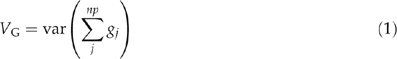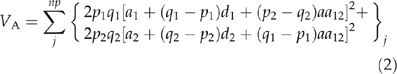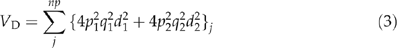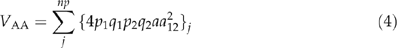where VG is the total genotypic variance, np is the total number of pairs of loci, gj is the individual genotypic value at each pair of loci, VA is the additive variance (variance of breeding values), VD is the dominance variance (variance of dominance deviations) and VAA is the additive-by-additive variance, a1 and a2 are the additive effect of loci 1 and 2, d1 and d2 are the dominance effects, and aa12 is the additive-by-additive effect of the pair (1 and 2). The gene frequencies of alleles A, B, a and b are p1, p2, q1 and q2, respectively. All additive-by-dominance and dominance-by-dominance genetic effects are ignored. Fuerst et al. (1997) also derived genetic covariance components between and within loci, but they are omitted in this study.

### Model 2

The second model (Model 2) was originally proposed by Cockerham (1954) and further developed by Kao and Zeng (2002) and Zeng et al. (2005). The main idea of this model is to define the genotypic value by using the orthogonal contrast scales to partition the genetic variance components, and hence, create a genetic model for modeling multi-locus interactions in a population (Cockerham, 1954). The partition of the variance components with the orthogonal contrasts is useful for expressing the correlation between relatives including interaction effects.

The orthogonal contrasts are used for partitioning the sum of squares in a one-way analysis of variance (ANOVA), and have to fulfill the following conditions for the two-locus case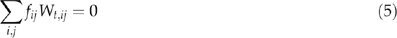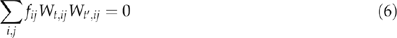where index t refers to the t: th contrast, index i and j refers to the genotype at locus 1 and 2, respectively. The index i=2, 1, 0 corresponds to the genotype AA, Aa or aa, fij is the frequency of genotype ij and Wtij is the orthogonal contrast of genotype ij. The first condition is to assure that deviations around the mean are considered, whereas the second condition is to check that the contrasts are orthogonal (the inner product of the contrasts are zero). The allele frequency at one locus is assumed to be uncorrelated with the frequency at the other locus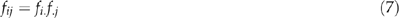where a dot refers to the marginal (or averaged) genotypic frequency at that locus.

The different variance components are functions of genotypic frequencies and genotypic values, and in the two locus case the variance component σt2 corresponding to the scale Wt formulated as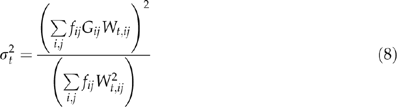where Gij is the genotypic value of genotype ij. For each locus, the orthogonal contrast can be divided into a linear and a quadratic term, which refer to an additive and a dominance genetic effect, respectively. The multi-locus interactions can be partitioned into second degree (additive-by-additive epistatic interaction), third degree (additive-by-dominance and dominance-by-additive interactions) and fourth degree terms (dominance-by-dominance interaction). In Supplementary Table 2, the orthogonal scale Wt is expressed depending on the genotypic value of an individual in a two-locus case (t=1,…,8).

Kao and Zeng (2002) expanded Equations (5), (6), (7) and (8) to cover the multi-locus situation by summing over all loci and adding all contributing terms. This gives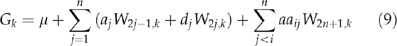where Gk is the genotypic value of genotype combination k, μ is the average genotypic value in the population, aj the additive effect of locus j, dj the dominance deviation of locus j, aaij the additive-by-additive epistatic effect of loci combination ij and n the number of loci used in model. The interaction terms in this study are restricted to include only second-order terms, because the number of interaction terms soon becomes very high when the number of loci (n) increases. For Model 2, it is easy to capture all second-order interactions between all loci by adding ad, da and dd interaction components, but this feature was omitted in this work as Model 1 and Model 2 were to be compared. Throughout the simulations, free recombination was assumed for loci both within and between chromosomes in Model 2. See Cockerham (1954), Kao and Zeng (2002) and Zeng et al. (2005) for further details concerning Model 2.

### Genetic and environmental parameters

Each individual was assigned a genotypic value according to Supplementary Table 1 or Equation (9) depending on the genetic model examined. Initial broad sense heritability was set to 0.3, and used for generating environmental standard random numbers for each individual according to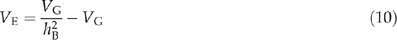where hB2 is the broad sense heritability (Falconer and MacKay, 1996). In order to investigate whether the selection response is affected by non-additive gene action, the initial ratio of the non-additive genetic variance and the additive genetic variance was set to a high value (Table 1). The values of the additive, dominant and epistatic effects were tuned based on some preliminary simulations.

### Mating and selection procedures

A random mating base population was created in order to satisfy Hardy–Weinberg equilibrium for all loci. We assume that no mutation, no migration, no selection and no stochastic effects resulting from genetic drift exist in the base population. Two alleles at each locus were randomly assigned giving an initial allele frequency of 0.5. Also, simulations with initial genotype frequencies of 0.05 and 0.95 for the favorable homozygote (i.e. f(AA)=f(BB)=0.05 and f(AA)=f(BB)=0.95 in the case of two loci) were performed. In each generation, offspring were produced by random mating for a period of 10 generations (resulting in a base population of 150 individuals). Each mating resulted in one surviving offspring.

When the base population had been established, phenotypic truncation selection was applied. The 100 individuals with the highest phenotypic values (s=1/3) were selected for mating each generation during 15 generations of truncation selection. During each generation, genetic, additive, dominance and epistatic variances were estimated, as well as other genetic and population parameters (selection differential, selection response, average phenotypic value and average allelic frequencies). The selected individuals were mated at random to produce 50 new individuals, thus the population size was constant over the generations. Sex and year effects as well as genotype–environment interactions are considered to be absent in the model. Overlapping generations was allowed when directional selection was applied, whereby each individual was capable of producing offspring for five generations.

A Monte–Carlo method was used to simulate the population undergoing selection in order to generate the median of the genetic variance components and to obtain a 95% confidence interval. Simulations were repeated 1000 times for each set of genetic parameters. Fifteen generations of selection were simulated for both Model 1 and 2.

## Results

### Base population

In order to exclude the possibility that the genetic variance components in the base population were affected by genetic drift, we examined the evolution of the additive variance (VA) for different genetic models over 10 non-overlapping generations. We examined VA using Model 2 for all configurations of gene action in the case of four loci. The development of VA in the base populations showed no signs of any drift effects and was roughly constant in all gene modes. We also performed simulations with 1500 individuals in the base population where median of variance components and 95% confidence intervals were computed and compared to the original 150 individual base population. There was no evidence of any deviations of the median level of the additive variance in the original 150 individual base populations.

### Model 1

As expected, when pure additive gene action (A) affected the trait, VA and VG decreased when selection was acting on the population until fixation of the desirable genotype was reached (Figure 1a).

Both the A/D− and A/AA regimes increased VA during the initial five generations (Figure 1b and c), but the increase of VA was greater in the A/AA case, where the maximum level of additive variance (VAmax) was 273% of the initial VA at generation 5. For A/D−, 195% of the original VA was present at generation 5. These differences can be attributed to the effects of interacting genes, which increase the genotype value of the favorable homozygote (Supplementary Table 1). In the A/D/AA mode, VA increased slightly, but the strong overdominance in the model gave a weaker increase in VA (Figure 1d) compared to the other modes, where non-additive variances were present. However, the greatest increase in VA occurred in the A/D−/AA case where VAmax was 490% of the initial level at generation 6 (Figure 1e).

The level of VA in the population over 15 generations of selection varied between the models. The A/D/AA case had the highest ratio of remaining additive variance. Simulations including gene action A/D−, A/AA and A/D−/AA reached fixation of the favorable genotype before the simulations ended (and therefore no additive variance was left in the population at generation 15). In all simulations where non-additive gene action was included, both VD and VAA decreased steadily. It can be seen in Equation (3) for VD and in Equation (4) for VAA, that the highest non-additive genetic variance will occur at intermediate level of allele frequencies (p1=p2=q1=q2=0.5).

In Supplementary Figure 1a–e, the additive variance surface is plotted as a function of allele frequencies for gene action A, A/D, A/AA, A/D/AA and A/D−/AA. Supplementary Figure 1a confirms the results obtained from the simulations where VAmax occurred at an intermediate level of allele frequency for the A mode. In case of the A/D− mode, VAmax occurred when p1=p2=0.78 (Supplementary Figure 1b). The level of VAmax is greater in the case of A/D− mode compared to pure A mode, which is confirmed by the simulations (Figure 1a and b). In the A/AA mode, VA has two maxima that occur when p1=1, p2=0.5 and p1=0.5, p2=1 (Supplementary Figure 1c). Also, in A/D/AA mode VA have two conditional maxima (p1=1, p2=0.32 or p1=0.32, p2=1; Supplementary Figure 1d). However in the case with two maxima, VAmax cannot be utilized by phenotypic selection because both loci contribute equally to the trait expression, which will result in similar allele frequencies at both loci during the progress of selection. VAmax occurred when p1=p2=0.82 for gene action A/D−/AA (Supplementary Figure 1e). This peak can be utilized in the selection process, and therefore explain the great increase in VA (Figure 1e). When the variance surfaces for the two full models are compared to the simulation results (Supplementary Figure 1d–e), it can be concluded that the additive variance surfaces differ considerably in shape depending on the direction of the dominance effect. For Model 2, it is difficult to calculate the variance surface plots without constraining the proportions of homozygotes and heterozygotes in the population.

### Model 2

In the case of two loci, Model 2 gave similar results to Model 1: for the A mode, VA decreased as predicted (Figure 2a). When non-additive gene action were present, VA increased for modes A/D−, A/AA, A/D/AA and A/D−/AA (Figure 2b–e), but in the case of A/D/AA the increase was very small and lasted for only the first generation of selection.

The number of loci used in the simulations of Model 2 greatly affected the genetic variance components during generations of selection. When four loci explained the trait, as expected, VA decreased in mode A (Figure 3a). For the non-additive models, VA increased considerably more in comparison with the two-locus case (Figure 3b–e). Except for a slight increase in VD and VAA in the four locus case for some modes, the highest level of VD and VAA was found in the base population and decayed during selection until it vanished. However, in the two-locus case, VD and VAA had an almost identical development over time (Figure 2d and e).

VAmax occurred at different generations in the simulations and depended heavily on the number of loci used in the model (except for in case A, where VA decreased until fixation). For both A/D− and A/AA cases, two and six generations of selection passed until VAmax was reached for two and four loci, respectively. The level of VAmax compared to the initial VA was slightly higher in A/AA (Figure 3c) than in A/D− (Figure 3b), suggesting that VAA rather than VD generates a greater conversion into VA. In model A/D/AA, VAmax occurred after one and nine generations of selection in the two- and four-locus cases, respectively. For the A/D−/AA mode, 6 and 13 generations of selection passed until VAmax was reached in the two- and four-locus cases, respectively. Clearly, the A/D−/AA mode stands out, especially in the two-locus case, where the other models reached VAmax quickly. In the A/D−/AA case, a higher VAmax was obtained than in all other cases, both for two and four loci.

Figures 2 and 3d and e show that the direction of the dominance affected the development of VA. The increase in VA was greater when negative overdominance was present (A/D−/AA) than if positive overdominance was present (A/D/AA). This effect was most obvious in the case of four loci and is probably caused by homozygotes having an increased advantage compared to the heterozygotes in the selection procedure.

For the A mode, in the four-locus case (Figure 3a), the behavior of VA was initially more unstable than the two-locus case. However, after six generations of selection, VA stabilized and decayed as predicted by standard theory (Crow and Kimura 1970).

The selection response (measured as mean phenotypic standard units) is plotted in Figure 4. Clearly, if non-additive effects were present in the genetic make-up, the population had a greater long-term selection response compared to the plain A configuration. Hence, the phenotypic mean was also greater when selection was relaxed at generation 15 for the models that included non-additive effects. The difference in selection response after three generations of selection between the different gene configurations was marginal, suggesting that short-term selection is less sensitive to genetic configuration. The great response to selection in the A/D−/AA case could be explained by strong negative overdominance and by strong additive-by-additive epistasis that increases the level of additive variance, and hence, increases the selection response.

For A gene action, different number of loci yielded similar responses to selection. For A/D−/AA gene action however, the number of loci had great impact on the response. In the two-locus case, the increase in phenotypic value started to decay, while in the four-locus case, no such tendency could be seen. As more interactions could be kept for four loci, this configuration was expected to give a higher response to selection. Only the responses under the extreme gene modes (A and A/D−/AA) are displayed here.

## Discussion

We have implemented two different multi-locus genetic models and used them to simulate a population undergoing directional truncation selection. The models can incorporate dominance and epistasis and we evaluated the effect of non-additive genetic interactions on the genetic architecture after selection. We show that both the number of loci behind the trait and the presence of non-additive genetic effects greatly influenced the change in genetic variance components in populations subjected to selection. Hence, we argue that non-additive genetic variance can have a considerable impact on the response to both natural and artificial selection, and a one-sided focus on additive genetic components in conservation and breeding oriented projects is unwarranted.

### Multi-locus genetic models

Previously, most simulation studies have examined pair-wise interactions between loci (Model 1 in this study) (e.g. Gill, 1965a, 1965b and 1965c; Young, 1966, 1967; Fuerst et al., 1997). The genotypic value is then obtained by summing over all pairs of loci. However, in the model proposed by Cockerham (1954) (Model 2 in this study), all interaction terms can be included when computing individual genotypic values.

Clearly, we can conclude that the release in additive variance is greatest in the case of larger numbers of loci (four) due to the greater number of second-order interactions between the loci involved. Naciri-Graven and Goudet (2003) reached a similar conclusion in a study of the consequences of population bottlenecks. Moreover, the additive variance is kept for more generations of selection giving an increased selection response over time.

As pointed out by Lynch and Walsh (1998), the number of interacting second-order terms increases very rapidly if there are many loci coding for a trait. If there are n loci, the number of possible two-factor epistatic interaction terms (A·A, A·D, D·A and D·D) is 2n (n−1). Hence, even though each two-factor term may be small, the summation of all terms could give a significant contribution to the genotypic value of an individual. This fact makes the number of loci very important when estimating genetic variance components in a population. Another complication concerning the number of loci in genetic models is that all loci usually are assumed to be unlinked, and therefore covariance terms between loci will disappear. However, this is an idealized situation and in the case of selection untrue because selection will induce linkage disequilibrium that will produce biased results, especially if large number of loci determines the trait and if inbreeding is present (Barton and Turelli, 2004).

We can conclude that the mode of gene action greatly affects the genetic variances in a population undergoing truncation selection. In all cases where non-additive variance was present in our simulations, the additive variance increased initially under selection, in a manner depending on the particular combination of the genetic interactions and the number of loci that were used. These results confirm earlier findings obtained by Fuerst et al. (1997) for Model 1 and by Carter et al. (2005) using different directions of the epistatic effect to simulate the evolvability of a quantitative trait.

The level and direction of the genetic effects used in the models will have an impact on the development of the additive variance component, and hence, the ability of the population to evolve and respond to selection. For example, the different directions of the dominance effect could either increase or diminish the additive variance in the population both in the two- and four-locus cases. Furthermore, the direction of the epistatic effect could influence the evolvability of the additive variance considerable (for more details, see Carter et al., 2005). For genetic drift, Willis and Orr (1993) and Goodnight (1988) emphasized that the additive effects in the population might change because of the induced change in allele frequency. The presence of non-additive genetic effects could also alter the genetic background for other genes and therefore also change the allelic effects. The existence of non-additive variance induces a complicated dependency between allele effects and frequencies, which makes it challenging to derive general analytical results. Walsh (2004) concluded that it is very difficult to make theoretical predictions for the long-term response to selection if only the genetic parameters of the base population are known. Hence, simulation studies appear to be the best way to study the effects of longer-term selection.

One of the results in this study was that the non-additive variance became exhausted after a period of selection and the increased selection response would eventually reach a plateau as indicated in Figure 4a–b. Unfortunately, we could not find many results in the empirical literature confirming this result, but Laurie et al. (2004) concluded that no non-additive variance was present after 70 generations of directional selection in Maize when examining the genetic architecture of oil content in the famous Illinois long-term selection experiment. This result contrasts with the findings of Carlborg et al. (2006), who found considerable amounts of epistasis between growth genes in chicken subjected to 42 generations of selection.

Additive-by-additive epistasis seems to induce a greater increase of additive variance than dominance variance when the initial amount of dominance and epistatic variance components are equal. This result has been found before both in truncation selection and in population bottlenecks simulations (Fuerst et al., 1997; López-Fanjul et al., 2002). Because epistasis is a second-order term, an increase in the number of loci could considerably change the outcome of selection on the genetic variance components. As the number of loci increases in the model, the number of possible between locus interactions will grow exponentially (Carlborg et al., 2006).

Walsh (2005) emphasized that for sexual diploid organisms, one important feature of additive-by-additive epistasis (and higher order additive epistasis terms) is that this effect could be transferred from parents to progeny as the effects arise due to the fact that one additive acting allele at one locus could be affected by another additive acting allele at another locus. As a result, the genetic covariance between parents and offspring could increase and hence, change the breeding values of the progenies. However, in random mating populations, this effect is reduced or even cancelled out by recombination among alleles that destroys favorable genotypic combinations.

Historically, epistasis often has been overlooked when estimating quantitative genetic parameters by including it in the residual error variance in the classical additive statistical model. This will lead to biased interpretations of the additive variance and could affect which individuals are selected in conservation and breeding programs. However, estimation of non-additive effects in experimental populations using classical quantitative theory is difficult (Lynch and Walsh, 1998; Carlborg and Haley, 2004).

### The effect of different initial gene frequencies

Even though the effect of different levels of initial allele frequencies was not the main topic of this study, we will briefly discuss the issue here. In most domesticated species subjected to artificial selection or natural species in small populations, the allele frequencies will not be at an intermediate level. Most probably, allele frequencies will deviate from equilibrium. Therefore, we performed additional simulations with the favorable alleles being either rare or frequent. When the initial frequencies of the favorable alleles were small, the values of the different genetic effects had big impact on the outcome of the selection procedure. Positive additive, dominance and epistatic effects resulted in a positive selection response and increased the additive variance component during selection. Clearly, the level of the additive variance was greater when non-additive variance was present compared to pure additive gene modes. However, strong negative dominance or positive additive-by-additive epistasis diminished the additive variance and fixed unfavorable alleles after only a couple of generations of selection. This phenomenon depends on the level of the different genetic effects and also on the initial starting allele frequencies. However, if selection based on genetic markers is deployed directly on the favored genes, this phenomenon might be avoided.

When the favored alleles were frequent, the selection process ended after one or two generations and the favored genotype became fixed. As the initial genetic variance was smaller in this case compared to the simulations with intermediate starting allele frequencies, the development of the variance components were very sensitive to the starting values and a lot of noise appeared, especially in the four loci version of Model 2 with epistasis.

### Comparison between model 1 and 2

Apart from the pure additive case, the development of additive variance differed between the models. In Model 1, the additive variance increased until generation five to seven (depending on which gene effects that were included in model) where it started to decrease. In Model 2, the greatest increase in additive variance occurred after the first selected generation. The total increase in additive variance was greater in Model 1. Another difference between the models was the level of remaining additive variance at generation 15. Because Model 1 showed a greater response to selection, less additive variance remained at generation 15. For some cases in Model 1, the high response resulted in fixation of the favorable genotype in the breeding population when negative overdominance and/or epistasis were present. However, the remarkable selection response in Model 1 could be biased owing to the fact that the covariance terms between different genetic effects were excluded in the genetic make-up. As the genetic variance components are orthogonal to each other in Model 2, but not in Model 1 for modest changes in gene frequency, Model 2 will give more reliable estimates of the additive variance components. The absence of the genetic covariance terms explains why the response to selection was greater in Model 1. When the change in gene frequencies due to selection starts to become significant, both models produce overestimates of the selection response. This issue is discussed more in detail in Kao and Zeng (2002).

Another important difference between the two models is the way the individual genotypic values are computed. In Model 1, the genotypic value is independent of the allele frequencies in the population (Supplementary Table 1), while Model 2 takes the population allele frequencies into account when calculating genotypic values (Equation 9). This partly explains the higher level of additive variance in Model 1, and hence, the greater response to selection.

### Possible biases in this study

After generation 15, the bias increased significantly owing to the fact that expectations of allelic frequencies reached extreme values, which gave diffuse results especially in the four-locus case (including both dominance and epistasis). When allele frequencies reach such extreme values, the assumptions of independence between loci will be violated because of linkage.

It should also be pointed out that covariances among different kinds of genetic effects could contribute to trait expression and this effect could potentially bias experimental results and complicate the understanding of the increase in additive variance components produced by the action of genetic drift (Barton and Turelli, 2004). In this study, negative covariance terms were omitted in Model 1, which may have resulted in an overestimation of the additive variance. Also, covariance terms between genetic effects at different loci were omitted. Generally, the contribution of higher order terms are negligible compared to the lower order terms, but it is unclear how genes interact in multiple-locus situations (Barton and Keightley, 2002; Carlborg and Haley, 2004).

Absence of inbreeding in this study is a simplification because the level of inbreeding will increase when selection is applied on a breeding population. In the case of inbreeding, the presence of non-additive variance could actually increase additive variance within selected lines (Walsh, 2005). Experimental evidence for this phenomenon has been found in inbred mice strains (Cheverud et al., 1999). However, the number of selected individuals per generation was relatively large in our simulations so the effect of inbreeding could be considered to be relatively small.

Moreover, the way the population is defined is important for the outcome of selection and genetic drift experiments. If data on many individuals are used in an overall pedigree structure, the change in genetic variance in the population may be negligible and hence, fit the infinitesimal model. But, if smaller family-based pedigrees are used, differences in genetic variance components could more easily be detected (e.g. Martinez et al., 2000). The change in genetic variances in this study would probably have been smaller if we had based our calculations on the whole pedigree, but to what degree is difficult to say.

## Conclusions

The additive variance of a trait in a population undergoing directional selection could initially be increased resulting in an increased heritability and, consequently, and increased response to selection. This behavior occurs when non-additive variance is present. Conversely, if only additive variance is present, the genetic variance will erode steadily until a plateau in phenotypic response to selection is reached and no further selection advances are possible.

Another important factor that influences the evolution of genetic variance components is the number of loci that code for the trait. If more complex interactions could be taken into account in the genetic architecture due to multi-locus interactions, more accurate predictions could be made in simulation experiments. Based on a large number of QTL mapping and expression studies, it is relatively clear that the infinitesimal model does not hold and that the number of loci that contribute to traits is relatively few. Some molecular genetic studies have also found substantial levels of dominance and epistasis. Hence, the findings from this study might be of considerable relevance for various areas in quantitative genetics.